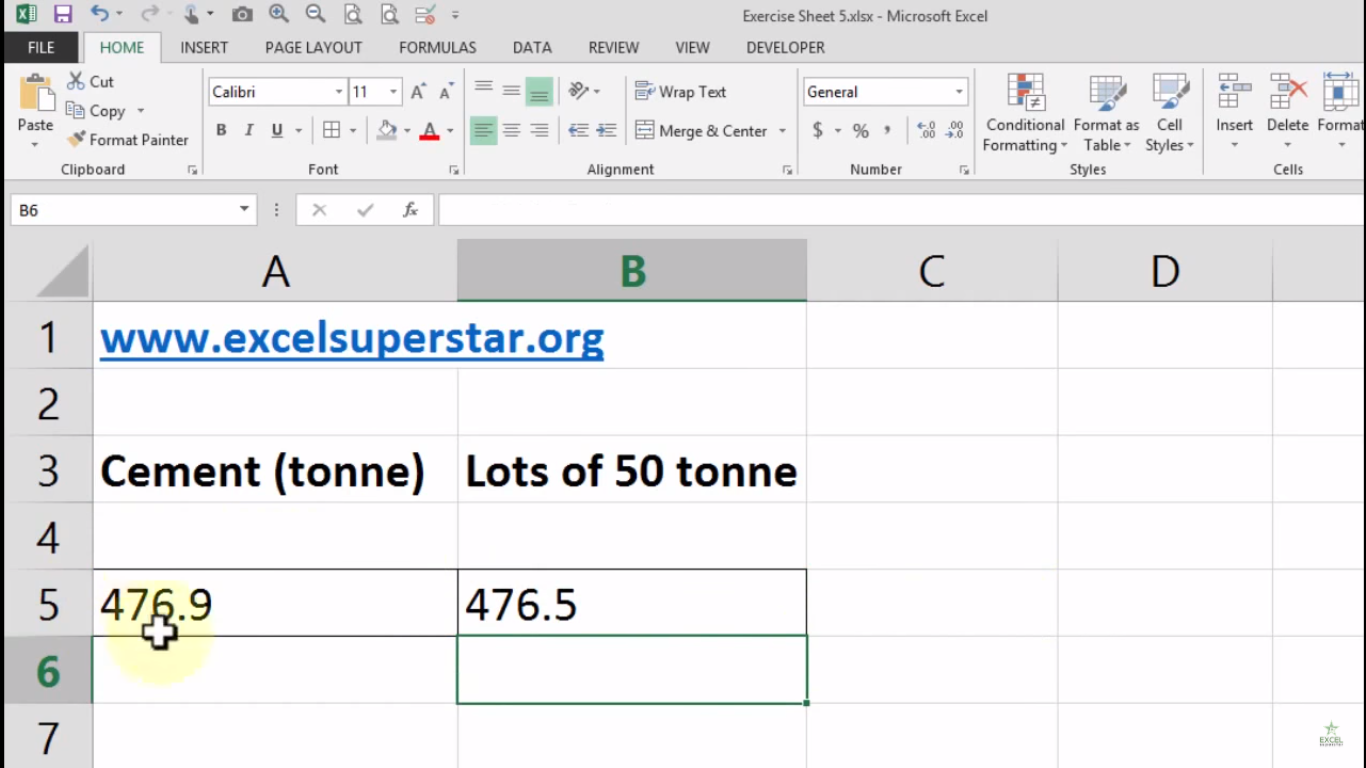# Excel Round FormulaHello Everyone In this tutorial we will be learning about Round Formula in Excel with the help of a question given below:

Question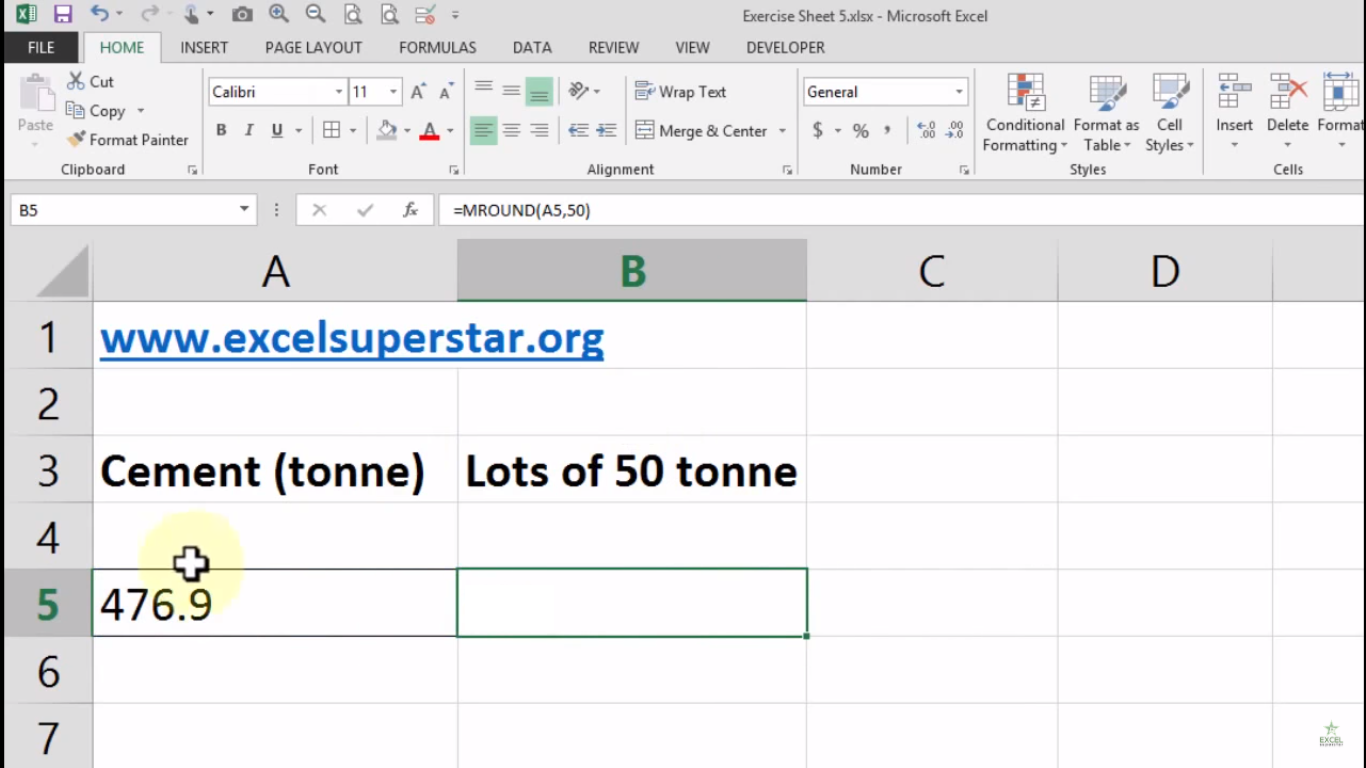In this table, I have a number denominated as 476.9, which represents cement production in (tonne).

Now I have to make a lot of 50 tonnes of cement to transport the batch. So how many tonnes I have to transport to complete a lot?

So to calculate the lots of tonnes which have to be transported we will be using ROUNDDOWN Formula

Note – You can also calculate the lots of tonnes using ROUNDUP Formula

Follow the steps given below in order to calculate it:

1. Press = ROUNDDOWN and open the bracket =ROUNDDOWN()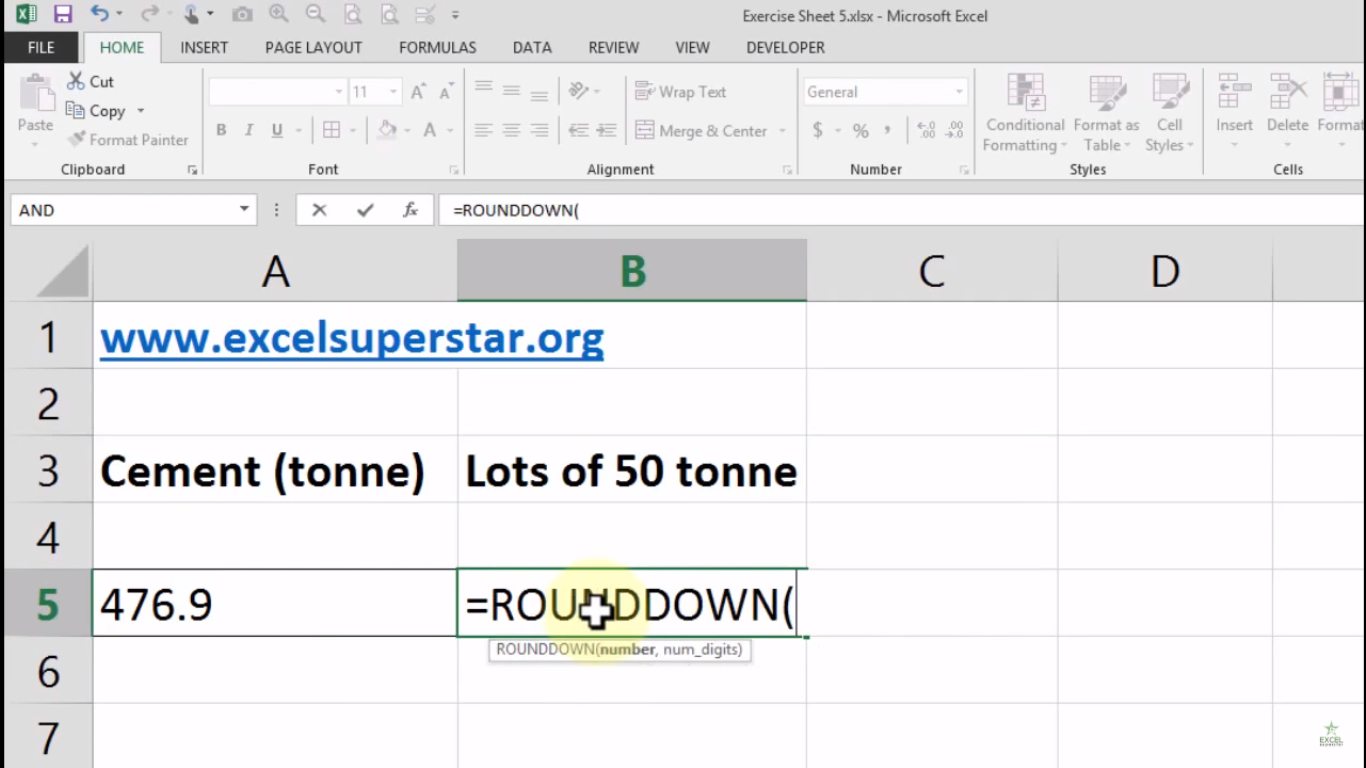2. Choose the Cement (tonne) cell =ROUNDDOWN(A5)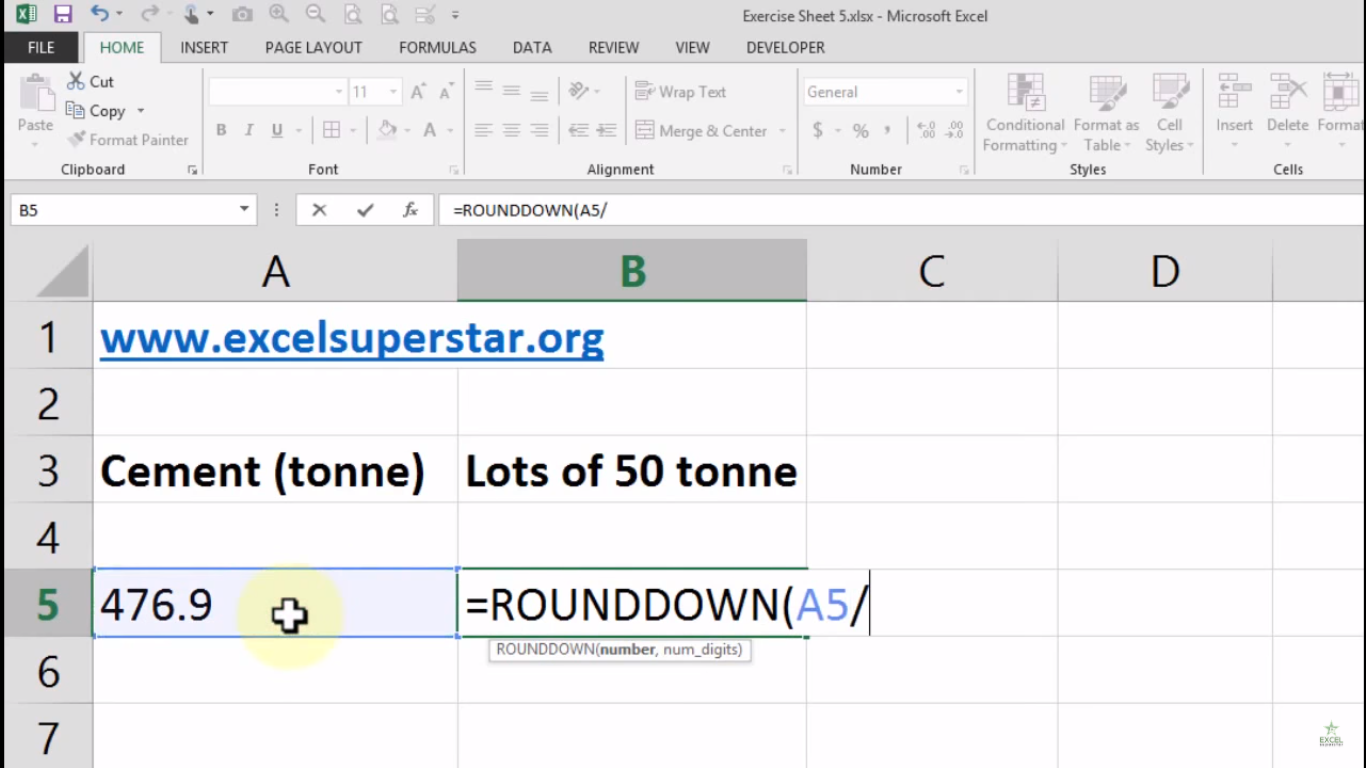3. Divide the cell by 50 and after that press comma (,) =ROUNDDOWN(A5/50)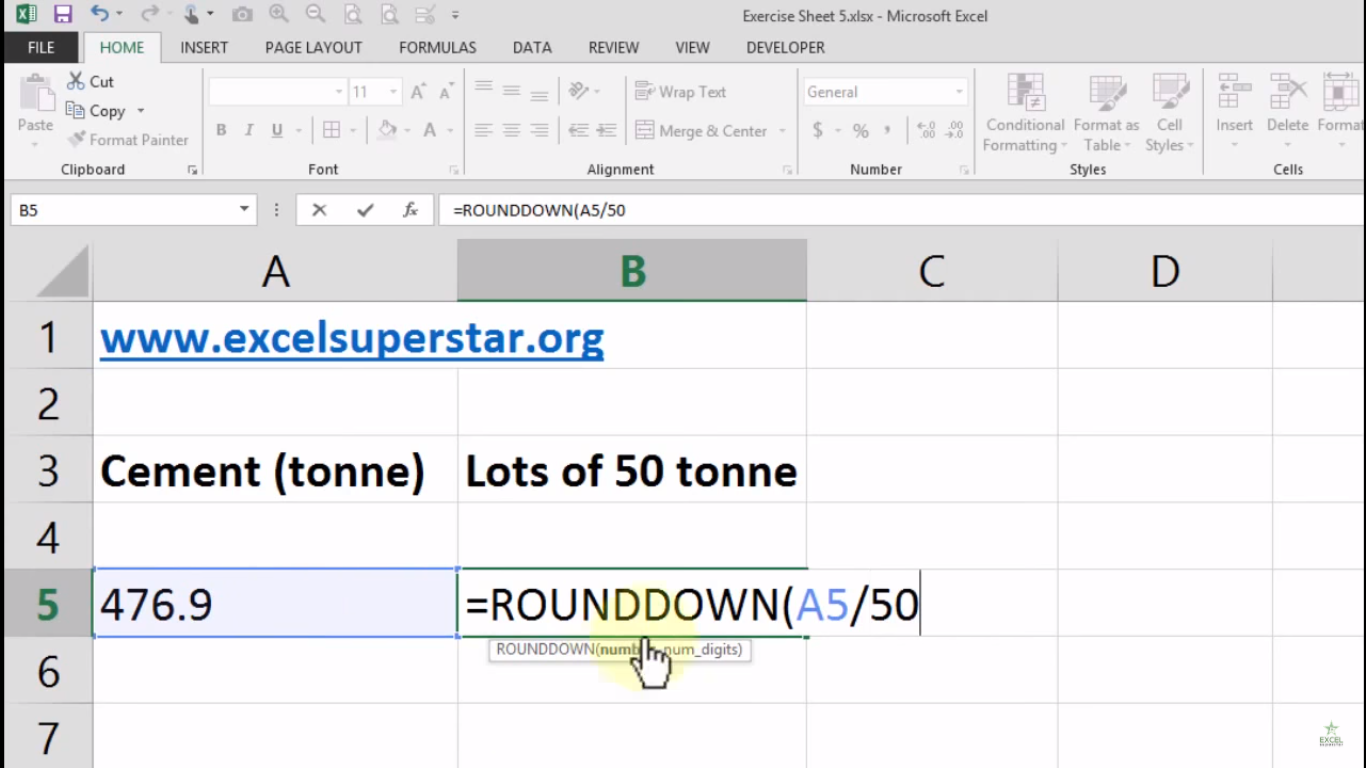4. Write 0 and close the bracket =ROUNDDOWN(A5/50,0)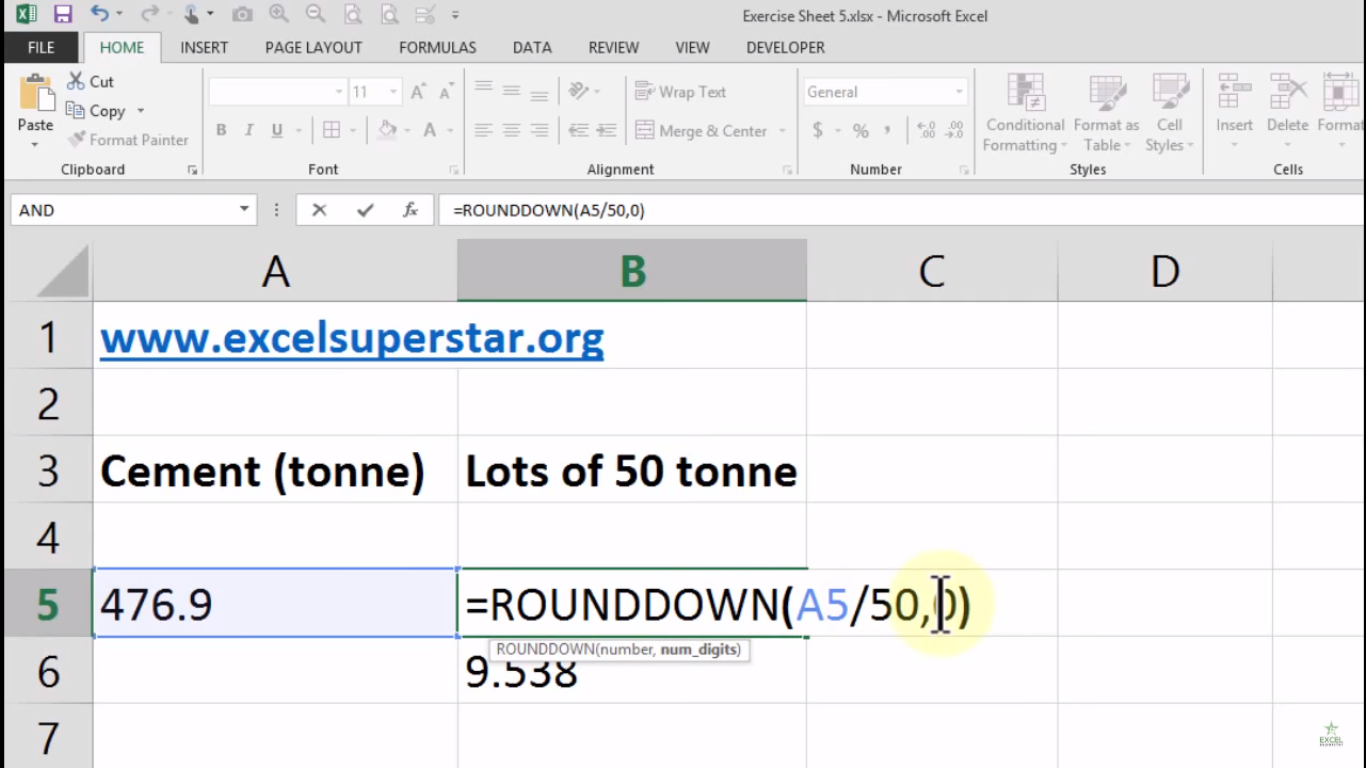5. Multiply from the same number from which you have divided the cell
=ROUNDDOWN(A5/50,0)*50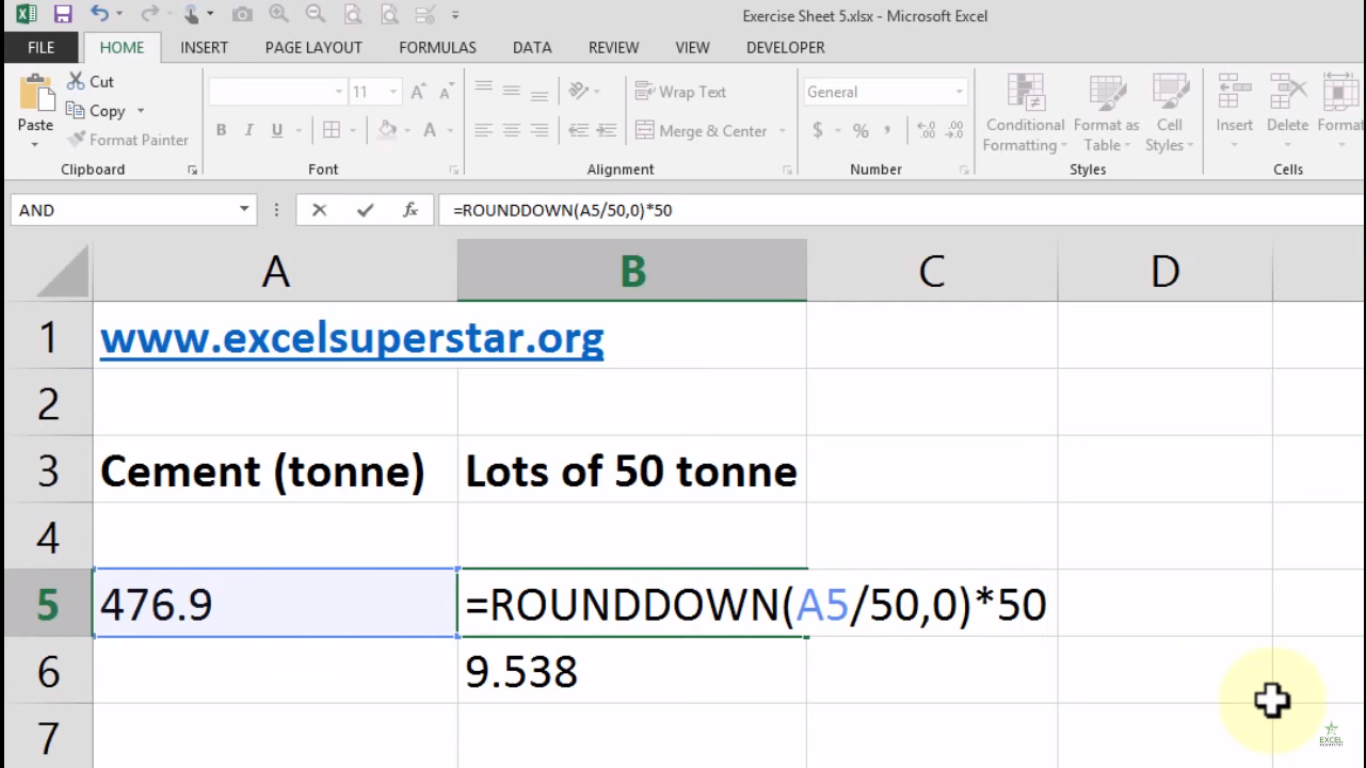6. Press Enter

7. Your answer will be 450 means you can transport 450 lots of tonne and the remaining 26.9 lots of tonne you can send in the next transport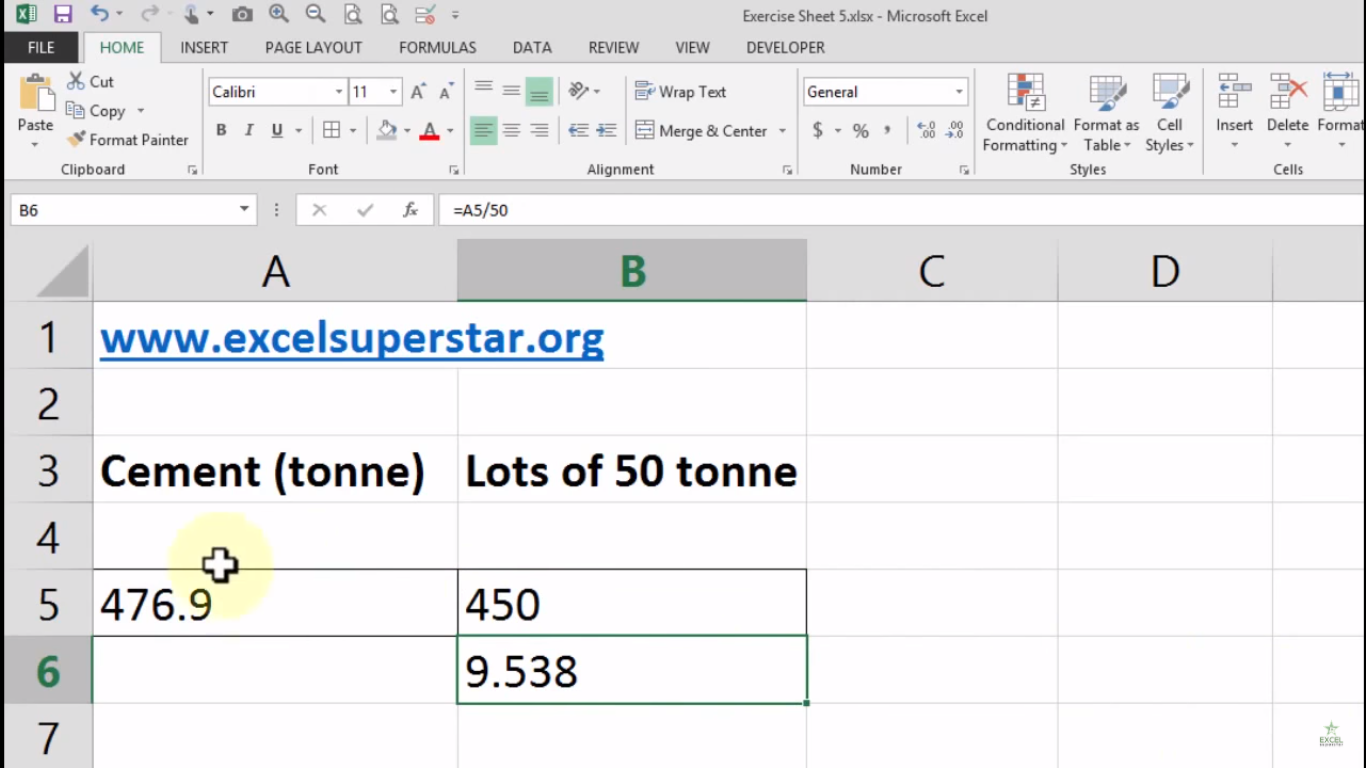Note – You can divide this by any number to calculate it

1. Take the Formula =ROUNDDOWN(A5/50,0)*50

2. Replace the number from 50 to 0.5 =ROUNDDOWN(A5/0.5,0)*0.5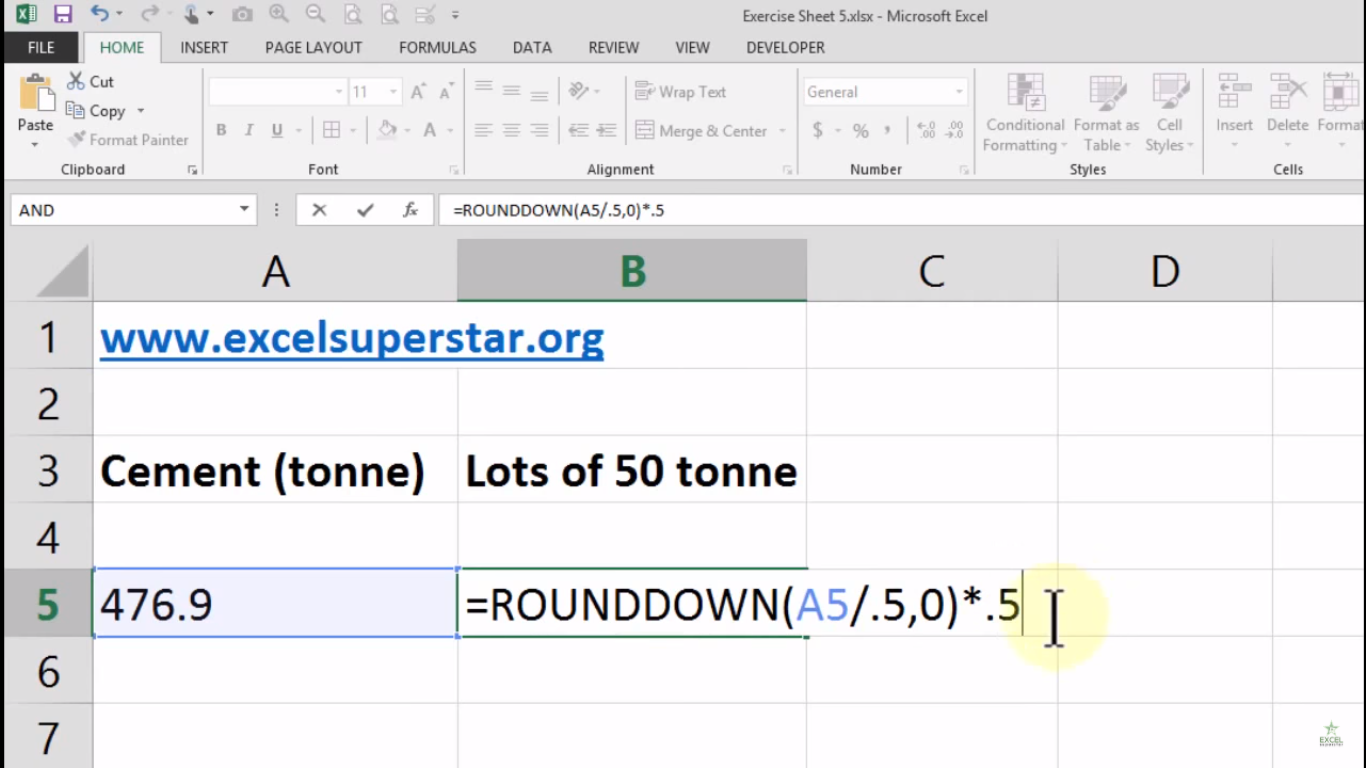3. Lastly press Enter and you will get your answer as 476.5 which means you can send 476.5 lots of tonne